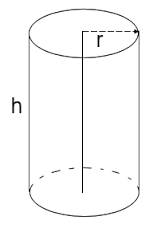### Cylinder Calculator

Calculate the various properties of a cylinder like like volume, curved surface area(CSA) and total surface area(TSA) for given values.Volume of Cylinder: [ πr²h ] Enter the radius = Enter the height = Volume of Cylinder =Curved Surface Area(CSA) of Cylinder: [ 2πrh ] Enter the radius = Enter the height = Curved Surface Area(CSA) of Cylinder =Total Surface Area(TSA) of Cylinder: [ 2πr(h+r) ] Enter the radius = Enter the height = Total Surface Area(TSA) of Cylinder =